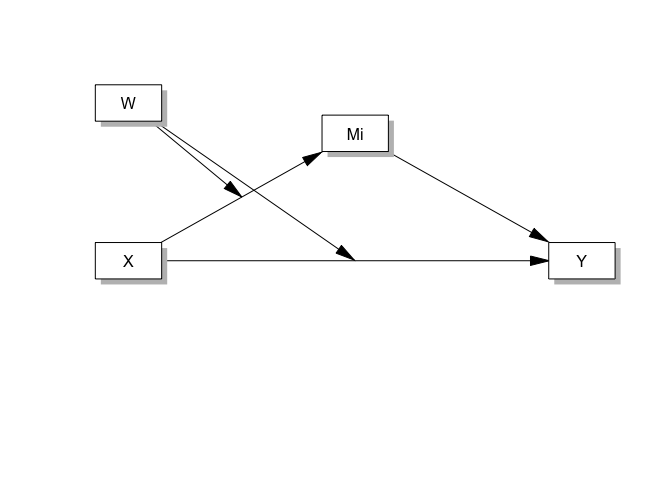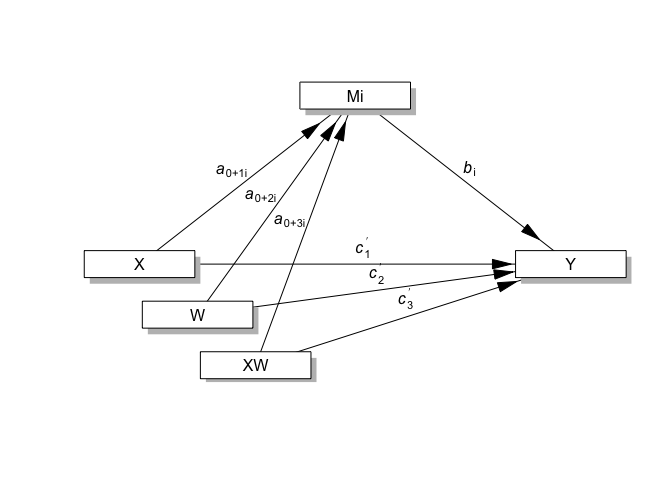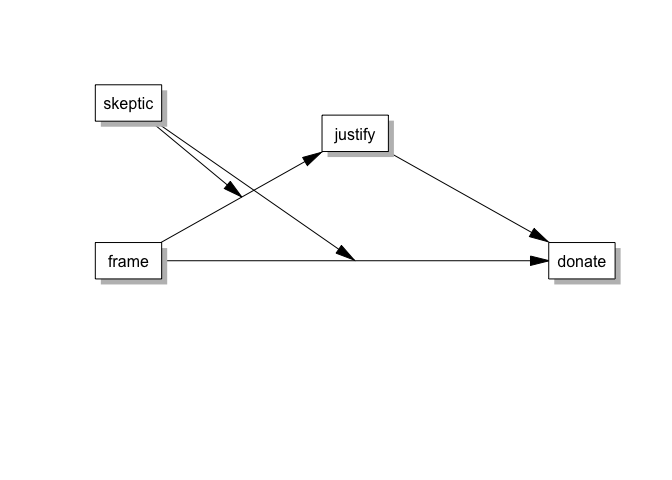# R package processR

#### 2020-01-27

The processR package aims to be a user-friendly way to perform moderation, mediation, moderated mediation and moderated moderation in R. This package is inspired from famous PROCESS macro for SPSS and SAS created by Andrew Hayes.

## PROCESS macro and R package processR

Andrew F. Hayes was not involved in the development of this R package or application and cannot attest to the quality of the computations implemented in the code you are using. Use at your own risk.

## Installation

You can install the processR package from github.

if(!require(devtools)) install.packages("devtools")
devtools::install_github("cardiomoon/processR")

## What does this package cover ?

The processR package covers moderation, mediation, moderated mediation and moderated moderation with R. Supporting models are as follows.

library(processR)
sort(pmacro\$no)
   0.0  1.0  2.0  3.0  4.0  4.2  4.3  5.0  6.0  6.3  6.4  7.0  8.0  9.0 10.0
 11.0 12.0 13.0 14.0 15.0 16.0 17.0 18.0 19.0 20.0 21.0 22.0 23.0 24.0 25.0
 26.0 27.0 28.0 29.0 30.0 31.0 35.0 36.0 40.0 41.0 45.0 49.0 50.0 58.0 58.2
 59.0 60.0 61.0 62.0 63.0 64.0 65.0 66.0 67.0 74.0 75.0 76.0

Currently, 57 models are supported.

## Example: Moderated Mediation (PROCESS macro model 8)

I will explain functions of processR package by a example.

## Concept Diagram and Statistical Diagram

You can draw concept diagram and statistical diagram easily. For example, you can draw the concept diagram for PROCESS macro model 8.

pmacroModel(8)You can draw statistical diagram of this model.

statisticalDiagram(8)## Make model equation for analysis

This package uses lavaan and mediation packages for analysis. The lavaan package is extremely customizable and can also model latent variables if your measurement model requires it. But it is difficult to figure out the model equation for analysis. You can make model equation easily. For example, if you want to perform moderated mediation with data disaster with the following concept model.

labels=list(X="frame",M="justify",Y="donate",W="skeptic")
pmacroModel(8,labels=labels)There is one moderator skeptic in this model. The moderating site is “a” and “c”. You can make model equation by the following command.

moderator=list(name="skeptic",site=list(c("a","c")))
model=tripleEquation(X="frame",M="justify",Y="donate",moderator=moderator)
cat(model)
justify~a1*frame+a2*skeptic+a3*frame:skeptic
donate~c1*frame+c2*skeptic+c3*frame:skeptic+b*justify
skeptic ~ skeptic.mean*1
skeptic ~~ skeptic.var*skeptic
CE.XonM :=a1+a3*skeptic.mean
indirect :=(a1+a3*skeptic.mean)*(b)
index.mod.med :=a3*b
direct :=c1+c3*skeptic.mean
total := direct + indirect
prop.mediated := indirect / total
CE.XonM.below :=a1+a3*(skeptic.mean-sqrt(skeptic.var))
indirect.below :=(a1+a3*(skeptic.mean-sqrt(skeptic.var)))*(b)
CE.XonM.above :=a1+a3*(skeptic.mean+sqrt(skeptic.var))
indirect.above :=(a1+a3*(skeptic.mean+sqrt(skeptic.var)))*(b)
direct.below:=c1+c3*(skeptic.mean-sqrt(skeptic.var))
direct.above:=c1+c3*(skeptic.mean+sqrt(skeptic.var))
total.below := direct.below + indirect.below
total.above := direct.above + indirect.above
prop.mediated.below := indirect.below / total.below
prop.mediated.above := indirect.above / total.above

## Full vignette

You can see full vignette for model 8 at http://rpubs.com/cardiomoon/468602

## Shiny App

I have developed a shiny app. You can test the app at http://web-r.space:3838/processR. I will appreciate any comment.

## How to perform this analysis with shiny app

You can see how to perform this analysis at http://rpubs.com/cardiomoon/468600

## Sample powerpoint file

In the shiny app, you can download the analysis results as a powerpoint file. You can download the sample file model8.pptx - view with office web viewer.